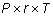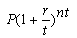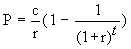﻿ 1.4 Calculation of Interestoffice (412) 9679367
fax (412) 967-5958
toll-free 1 (800) 214-3480

### 1.4  THE CALCULATION OF INTEREST

 I

nterest rates are quoted two ways: as simple interest and compound interest.

Simple Interest

Suppose you borrow \$1,000 for 1 year, and the bank charges you simple interest of 10%. At the end of the year, you will have to repay \$1,100.

If you borrow \$1,000 for three years, your total repayment will be \$1,000 + \$100 x 3. Here, the simple interest is the principal (\$1,000) times the interest rate (10%) times the number of periods (3). If you repay the loan in equal annual installments, you will make three payments of \$433.33.

Given the principal P (the amount borrowed), the interest rate, r, and the term of the loan, T, simple interest on the loan isIf you repay the loan in n installments, your payment per installment isNote that every period, the interest is calculated relative to the original amount borrowed.

Simple Interest Example

The simple interest on \$10,000 for six months at 8% per year is calculated in the following way. In one year, the simple interest is \$10,000 x 0.08 = \$800. As a result, in 6 months, the simple interest is \$10,000 x 0.08/2 = \$400.

In some cases, simple interest is computed for some specified number of days. Suppose you want to find out the simple interest for 137 days. In this case, you need to know the number of days in one year (or the "day count"). A banker’s year typically has 360 days. In some cases, the day count is 365 days or 364 days. Once the convention is known, the computation of simple interest is straightforward. With a day count of 360, the simple interest for 137 days is:

Simple interest = \$10,000 x 0.08 x (137/360)

Compound Interest

Suppose you borrow \$1,000 for 1 year and the bank charges you interest of 10%. At the end of the year, you will have to pay \$1,100. This is no different from simple interest.

The difference between simple and compound interest arises with multiple periods. Consider a two-year loan in which you only pay back the loan at the end of two years. Then, you would have to pay \$1,000 (1 + 0.10)2, which is \$1,210. What happens here is that at the end of one year, your new principal is \$1,100 = \$1,000 (1 + 0.10). The interest for the second year is calculated on this new principal.

Table 1.1 summarizes the repayment if you make only one payment on the loan. As you can see, you pay more if interest is compounded. This is because in Period 2, interest is charged on both the original principal and on the Period 1 interest.

 Simple Interest Compound Interest Principal \$1,000 \$1,000 Year 2 Payment \$1,200 \$1,210

Table 1.1 Simple versus Compound Interest

Therefore, compounding works against you if you pay off a loan only at the end. Compounding works in your favor, however, if you make intermediate payments. With simple interest, you would pay \$600 at the end of Year 1 and \$600 at the end of Year 2.

With compound interest, the story is more complicated, and involves the concept of amortization. Suppose you pay C at the end of each year. The interest on P is rP, so the amount C - rP is applied toward reducing the principal. Therefore, your principal remaining after one payment is P1 = P - (C - rP). The second year’s interest is now calculated on P1 , and is rP1, which is less than rP.

Here, you are paying less interest in the second year than you would with simple interest if C > rP.

You may be asking, what is C? It is determined by applying the principle of discounting future cash flows.

For the bank, P today must equal the present value of C in one year and C in two years:For our example, P = \$1000 and r = 0.10, so C = \$576.19. You can see immediately that C is less than the \$600 you would pay with simple interest.

Let us check our calculations at the end of Period 1. At this point, we have a one-period loan with principal amount P1 = P - (C - rP) = \$523.81. At the end of Period 2, C must cover this principal plus the interest on this principal (i.e. it must be the case that C = P1 (1 + r) = \$523.81(1.01) = \$576.19).

The process of recalculating the principal every time you make a payment is called amortization.

The Compounding Frequency

With compound interest, you have to be careful to consider not only the quoted interest but also the compounding frequency. Interest is usually quoted on an annualized basis with the compounding frequency stated separately (e.g. daily, monthly, quarterly, semi-annually, or annually).

If the quoted interest rate is r, compounded n times a year, interest per period is computed using the rate r / n. Therefore, if you invested \$1,000 for one year at 12% compounded monthly, at the end of the first month your investment would be worth \$1,000 x (1 + 0.01). At the end of two months, it would be \$1,000 x (1 + 0.01)2 . At the end of the year, it would be worth \$1,000 x (1 + 0.01)12 = \$1,126.825.

Table 1.2 shows the effect of the compounding frequency on your investment.

 Compounding Frequency Value Daily \$1127.48 Weekly \$1127.34 Monthly \$1126.82 Semi-Annually \$1123.60 Annually \$1120.00

Table 1.2 Effect of Compounding Frequency

As you can see, the greater the compounding frequency, the greater the final value, even though the quoted interest rate is the same. The moral of the story is: If you are investing money, then you want frequent compounding. If you are borrowing money, you want the frequency to be as small as possible.

The General Form

If interest is compounded t times per year, the investment lasts for n years, then in general, if P is the principal, then at the end of n years, the value isIn the limit, as t approaches infinity, the compounding interval approaches zero. This is called continuous compounding. This limit is:where e is the transcendental number = 2.718... .

A \$1,000 investment continuously compounded at 12% leads to a value of \$1,127.50 at the end of one year, which is only slightly greater than the value with daily compounding.

For most bonds, interest is calculated using compound interest, rather than continuously compounded interest.

The Annuity Formula

Many financial transactions involve a constant stream of payments over time. An ordinary annuity is a sequence of equal cash flows occurring regularly, say, every month or every year. Coupon payments from a bond and mortgage payments on a fixed-interest loan are examples of ordinary annuities.

The present value of an annuity is given by the annuity formula. This formula says that if you receive C every period for t periods, and the interest rate is r, then the present value of the annuity, P, isYou can see the derivation of the annuity formula in the Appendix to this chapter.

Ordinary annuity tables are often constructed for the case where C = \$1. These tables present the numbers pre-computed for this case in a table of interest rates r, by life t.

To value a t-period annuity in-advance, you add C and subtract one period from t in the ordinary annuity formula. To see why, consider the following example.

In Pennsylvania, the major winner in a Lotto game receives the cash prize as an annuity in-advance. For marketing purposes, the prize is quoted in total nominal dollars (e.g., \$21 million), but this prize is paid over 21 years. Quoting prizes in nominal dollars clearly sounds more favorable than quoting prizes in present dollars.

Suppose you are lucky enough to win \$21 million dollars paid at the rate of \$1,000,000 per year starting from the present. What is the present value of this prize (before tax)?

You can value your win by breaking the prize into two parts: \$1,000,000 today and an ordinary annuity of \$1,000,000 for 20 years. The present value of the first part is \$1,000,000, because \$1 today is worth \$1. If the market rate of interest is 8.15%, then by applying the annuity formula, the present value of this ordinary annuity component is worth \$9,709,515. Therefore, the present value of this annuity in-advance is the sum of the two parts, which equals \$10,709,515.

This is a lot less than the nominal dollar quote of \$21,000,000, but you might argue that "If I won the lottery I wouldn’t care about nominal versus present values!" After you work through The Lotto Case presented in the next topic, however, you may want to reevaluate this argument.